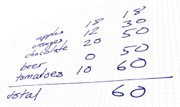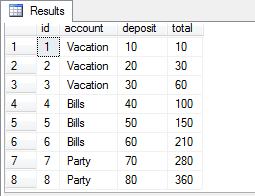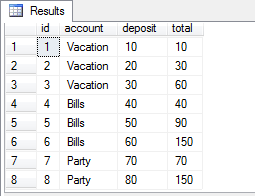# Calculate a running total in SQL Server 2012In the previous versions of SQL Server, calculating a running total for say, a bank account ledger, has been a frustratingly complex task. Fortunately SQL Server 2012 makes this a breeze with new support for windowed aggregate functions.

In this test example we’ll be creating a running total for an imaginary ledger using the OVER clause with SUM (you can get the entire test script here):

``SELECT a.id, a.account, a.deposit, SUM(a.deposit) OVER (ORDER BY a.id) AS 'total'    FROM #TestData a    ORDER BY a.id;``You can even do a running total for each account separately using the PARTITION clause:

``SELECT a.id, a.account, a.deposit, SUM(a.deposit) OVER (PARTITION BY a.account ORDER BY a.id) AS 'total'    FROM #TestData a    ORDER BY a.id;``## SQL Server 2005 and 2008

For those still stuck on older versions of SQL Server, the solution is a little less straightforward. There are a lot of techniques, but I have found the best performance using a recursive CTE (Common Table Expression), a feature added in SQL Server 2005.

``;WITH cte AS (    SELECT id, account, deposit, deposit AS 'total'        FROM #TestData        WHERE id = 1    UNION ALL    SELECT a.id, a.account, a.deposit, cte.total + a.deposit        FROM cte JOIN #TestData a ON cte.id + 1 = a.id)SELECT * FROM cteOPTION (MAXRECURSION 32767);``

There is one caveat though, since CTEs can do at most 32767 recursions. So if you have more records than that, you must fall back on the old sub-select:

``SELECT a.*, (SELECT SUM(b.deposit) FROM #TestData b WHERE b.id <= a.id) AS 'total'    FROM #TestData a``